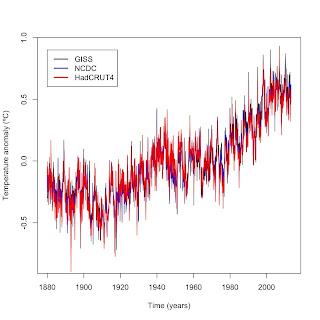### Meta-analysis

A meta-analysis is a technique that combines data from multiple sources into one large dataset for statistical analysis.  This overcomes one of the main problems with many studies, which is sample size.  It's difficult and expensive in many fields to get a sufficiently large sample.  Doing a meta-analysis on data from multiple studies is a powerful way to get around that limitation.

One possible use that I have yet to see in the published literature is using meta-analysis on global temperature data.  There are three surface temperature datasets (GISS, NCDC, and HadCRUT4), all of which are estimates of the true global temperature.  It should be possible to combine the three into a meta-analysis, which would have the added benefit of factoring out assumptions, internal errors, biases, etc, much like taking an average of the three would.

I downloaded GISS, NCDC, and HadCRUT4 data from their respective websites.  After downloading them, I imported each into R.  I first adjusted NCDC and HadCRUT4 to the same 1951-1980 baseline period as GISS by calculating their respective averages over the 1951-1980 time period then subtracting that average from the monthly data.  The end result of that process was this graph:Figure 1.  Graph of all three surface temperature datasets after adjusting them to the same baseline.
I then combined GISS and the baseline adjusted NCDC and HadCRUT4 into the same data frame and used the Reshape2 package to create a data frame with three variables: Time (in decimal year.month format), Dataset, and Temperature so that each month had three temperature estimates, one from each dataset.  Here's a brief view of that that dataset looks like:

 Time Dataset Temperature 1880 GISS -0.33 1880 HadCRUT4 -0.00614 1880 NCDC -0.1148 1880.08 GISS -0.27 1880.08 HadCRUT4 -0.11814 1880.08 NCDC -0.2498

I then analyzed the combined dataset using linear regression, time series analysis, and loess regression.

What's the benefit of going through all that work as opposed to analyzing each dataset separately?  More data per month means I can detect smaller trends with lower standard errors than I can with each dataset separately.  Here's an example using linear regression and the skeptics' favorite start point of 1998:

 Dataset Trend (ºC per year) Standard error p-value GISS 0.005967 0.002057 0.004183 NCDC 0.003367 0.001843 0.06932 HadCRUT4 0.003812 0.001960 0.05338 Meta-analysis 0.004382 0.001146 0.0001459

In the individual datasets, the linear trends for two out of the three datasets are not statistically significant.  The combined data, however, is statistically significant and the p-value is far lower than any single dataset.  How is that possible?  Note how the standard error is lower for the meta-analysis than for any of the three datasets individually?  There's the secret: Having 3x more data per month means that the estimate of the trend is more precise.  That's the power of a meta-analysis.  In this case, a meta-analysis shows that global warming did not stop in 1998 as skeptics claim.

### R Code

install.packages(c("reshape2")) #if you don't already have it
library(reshape2)